1、一对多查询

F6单元格输入以下公式：

=FILTER(A1:D11,A1:A11=G2)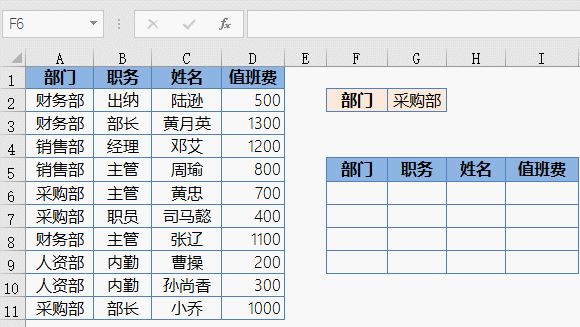FILTER函数的作用使用根据指定的条件筛选数据。用法是：

=FILTER(数组,条件,[没有符合条件的内容时返回的值])

=INDEX(A:A,SMALL(IF(\$A\$2:\$A\$11=\$G\$2,ROW(\$2:\$11),4^8),ROW(A1)))&""

2、多对多查询

F6单元格输入以下公式：

=FILTER(A1:D11,A1:A11&B1:B11=G2&G3)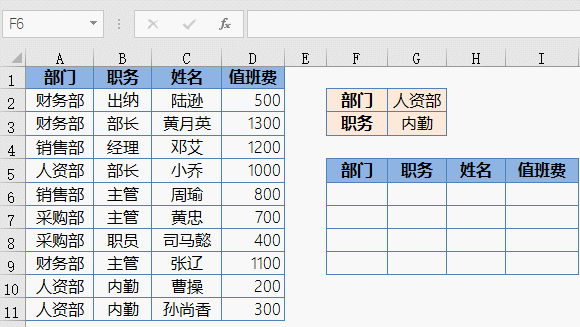=INDEX(A:A,SMALL(IF((\$A\$2:\$A\$11=\$G\$2)*(\$B\$2:\$B\$11=\$G\$3),ROW(\$2:\$11),4^8),ROW(A1)))&""

3、一列转多列

C2单元格输入以下公式：

=INDEX(A:A,SEQUENCE(11,5,2))&""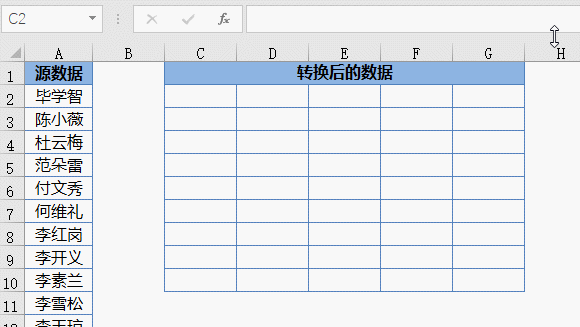SEQUENCE函数是专门用于生成序号的，使用起来特别简单，用法是：

=SEQUENCE(行数,[列数],[起始值],[步长])

=INDEX(\$A:\$A,ROW(A1)*5-4 COLUMN(A1))&""

4、提取不重复清单

G2单元格输入以下公式：

=UNIQUE(B2:B75)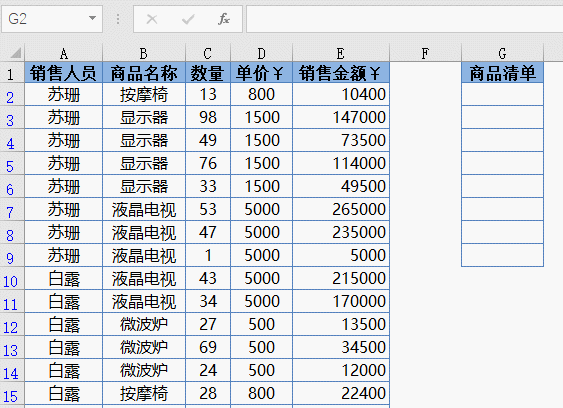UNIQUE函数的作用就是提取不重复值列表的。这个函数的完整写法是：

=UNIQUE(数据源,[按行/按列],[唯一值出现次数])

=INDEX(B:B,MATCH(0,COUNTIF(G\$1:G1,B\$2:B\$76),0) 1)&""

5、提取符合条件的不重复记录

F4单元格输入以下公式：

=UNIQUE(FILTER(D2:D17,A2:A17=G1))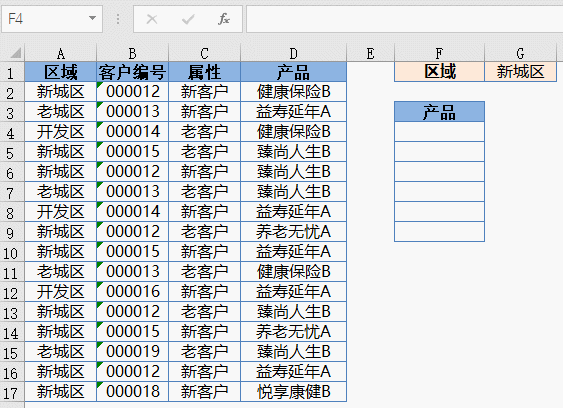=INDEX(D:D,SMALL(IF((MATCH(A\$2:A\$17&D\$2:D\$17,A:A&D:D,)=ROW(\$2:\$17))*(A\$2:A\$17=G\$1),ROW(\$2:\$17),4^8),ROW(A1)))&""